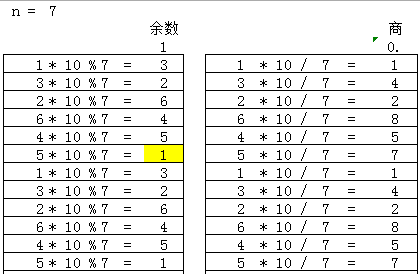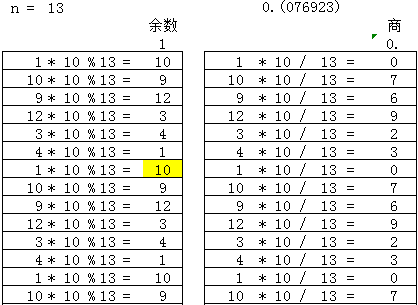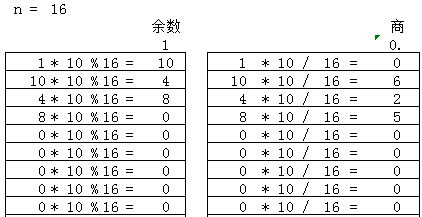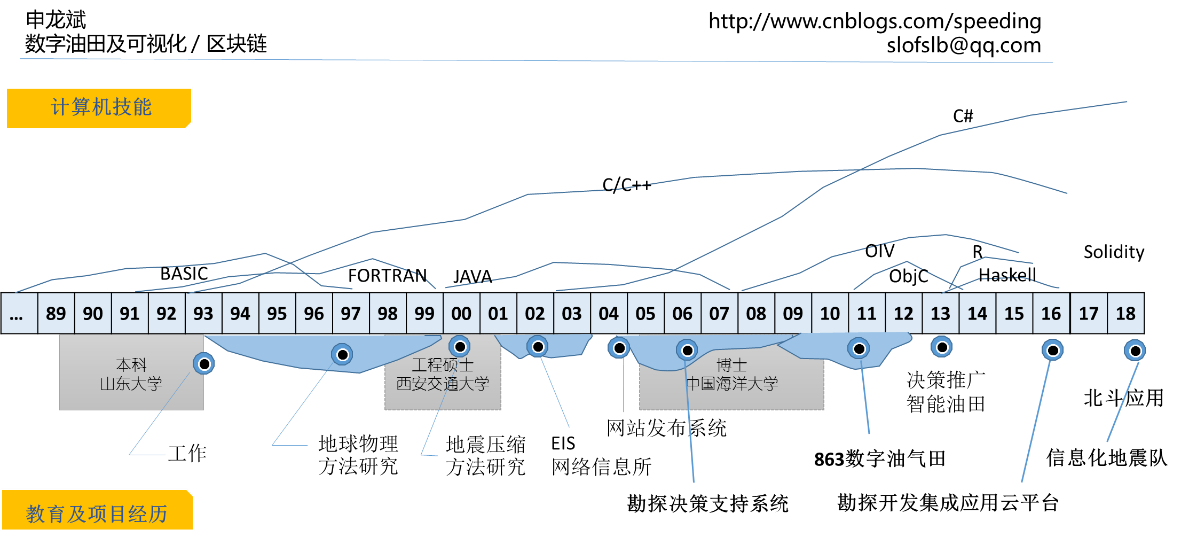# 用欧拉计划学Rust编程（第26题）

http://pe-cn.github.io/

## 第26题

1/6= 0.1(6) 表示0.166666…，括号内表示有一位循环节。

1/7= 0.(142857)，1/7有六位循环节。1）分子为1，表示一开始的余数为1

2）余数为0时，表示可以除尽，循环要终止

3）当余数重复出现时，表示找到了循环节，2个重复出现的位置就是循环节

fn reciprocal_cycle(d: u32) -> u32 {
let mut remainders : Vec<u32> = vec!; //余数
let mut digits : Vec<u32> = vec![]; //商

let mut numerator = 1; //分子
while numerator != 0 {
digits.push(numerator * 10 / d);
numerator = numerator * 10 % d; //余数
let pos = remainders.iter().position(|&x| x==numerator);
match pos {
Some(x) => { //余数重复出现时
return (digits.len() - x) as u32;
}
None => {
remainders.push(numerator);
}
}
}
0 //除尽的时候，表示循环节为0
}


let mut max_cycle: u32 = 0;
for n in 2..1000 {
let rc = reciprocal_cycle(n);
if rc > max_cycle {
println!("n={} cycle={}", n, rc);
max_cycle = rc;
}
}
println!("max reciprocal cycle: {}", max_cycle);


fn reciprocal_cycle(d: u32) -> u32 {
let mut remainders : Vec<u32> = vec!; //余数
let mut numerator = 1; //分子
while { numerator = numerator * 10 % d; numerator != 0} {
let pos = remainders.iter().position(|&x| x==numerator);
match pos {
Some(x) => { //余数重复出现时
return (remainders.len() - x) as u32;
}
None => {
remainders.push(numerator);
}
}
}
0 //除尽的时候，表示循环节为0
}


----==== Email: slofslb (GTD) qq.com 请将(GTD)换成@ ====----

---- 魔方桥牌象棋、游戏人生...